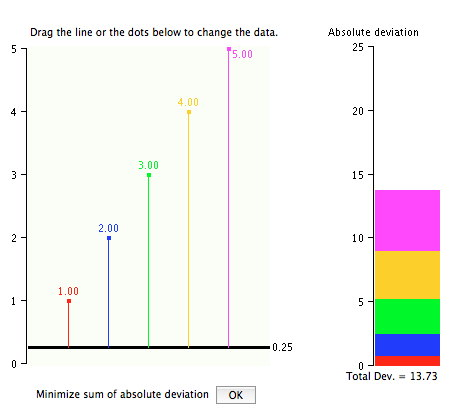# 3.5: Absolute Differences Simulation

•• David Lane
• Rice University
$$\newcommand{\vecs}{\overset { \rightharpoonup} {\mathbf{#1}} }$$ $$\newcommand{\vecd}{\overset{-\!-\!\rightharpoonup}{\vphantom{a}\smash {#1}}}$$$$\newcommand{\id}{\mathrm{id}}$$ $$\newcommand{\Span}{\mathrm{span}}$$ $$\newcommand{\kernel}{\mathrm{null}\,}$$ $$\newcommand{\range}{\mathrm{range}\,}$$ $$\newcommand{\RealPart}{\mathrm{Re}}$$ $$\newcommand{\ImaginaryPart}{\mathrm{Im}}$$ $$\newcommand{\Argument}{\mathrm{Arg}}$$ $$\newcommand{\norm}{\| #1 \|}$$ $$\newcommand{\inner}{\langle #1, #2 \rangle}$$ $$\newcommand{\Span}{\mathrm{span}}$$ $$\newcommand{\id}{\mathrm{id}}$$ $$\newcommand{\Span}{\mathrm{span}}$$ $$\newcommand{\kernel}{\mathrm{null}\,}$$ $$\newcommand{\range}{\mathrm{range}\,}$$ $$\newcommand{\RealPart}{\mathrm{Re}}$$ $$\newcommand{\ImaginaryPart}{\mathrm{Im}}$$ $$\newcommand{\Argument}{\mathrm{Arg}}$$ $$\newcommand{\norm}{\| #1 \|}$$ $$\newcommand{\inner}{\langle #1, #2 \rangle}$$ $$\newcommand{\Span}{\mathrm{span}}$$

Learning Objectives

• Learn which measure of central tendency minimizes the sum of absolute deviations

## Instructions

This demonstration allows you to examine the sum of absolute deviations from a given value. The graph to the right shows the numbers $$1, 2, 3, 4,$$ and $$5$$ and their deviations from an arbitrary starting value of $$0.254$$ (the figure displays this rounded to $$0.25$$).

The first number, $$1$$, is represented by a red dot. The deviation from $$0.254$$ is represented by a red line from the red dot to the black line. The value of the black line is $$0.254$$. Similarly, the number $$2$$ is represented by a blue dot and its deviation from $$0.254$$ is represented by the length of a blue line.

The portion of the graph with the colored rectangles shows the sum of the absolute deviations. The sum of the deviations is equal to $$0.746 + 1.746 + 2.746 + 3.746 + 4.746 = 13.73$$ as shown by the height of the colored bar.

In this demonstration, you can move the black bar by clicking on it and dragging it up or down. To see how it works, move it up to $$1.0$$. The deviation of the red point from the black bar is now $$0$$ since they are both $$1$$. The sum of the deviations is now $$10$$.

As you move the bar up and down, the value of the sum of absolute deviations changes. See if you can find the placement of the black bar that produces the smallest value for the sum of the absolute deviations. To check and see if you found the smallest value, click the "OK" button at the bottom of the graph. It will move the bar to the location that produces the smallest sum of absolute deviations.

You can also move the individual points. Click on one of the points and move it up or down and note the effect. Your goal for this demonstration is to discover a rule for determining what value will give you the smallest sum of absolute deviations. When you have discovered the rule, go back and answer the questions again.

## Illustrated Instructions

Below is a screen shot of the simulaton's beginning screen.Figure $$\PageIndex{1}$$: Beginning of the Simulation

Below is an example after the vertical line has been changed. The distances to the line have been recalculated.Figure $$\PageIndex{2}$$: Simulation after change

You can change the data by clicking on a data point and dragging. An example with changed data is shown below.Figure $$\PageIndex{3}$$: Simulation after changing data point

This page titled 3.5: Absolute Differences Simulation is shared under a Public Domain license and was authored, remixed, and/or curated by David Lane via source content that was edited to the style and standards of the LibreTexts platform; a detailed edit history is available upon request.